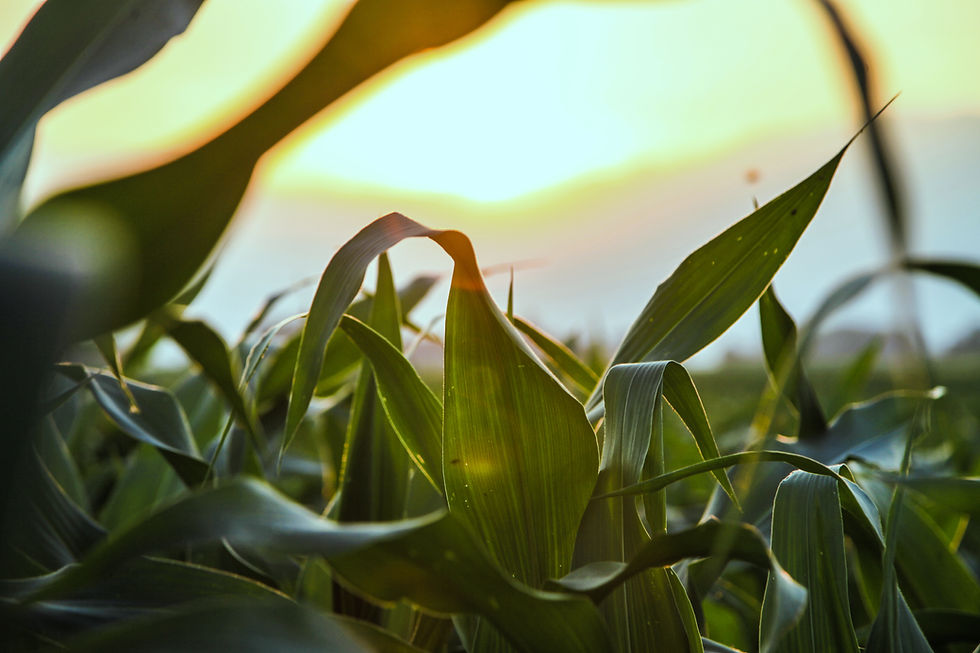# Combustion of hydrocarbons for AQA GCSE Chemistry1. Products and energy
2. Equations
3. Calculating energy change

Combustion is the burning of a fuel in oxygen. The products of combustion depend on oxygen supply to the fuel and whether the fuel contained any impurities. When there is incomplete combustion there is insufficient oxygen to convert the fuel fully into carbon dioxide.When combusting hydrocarbons the equation will depend on whether the oxygen supply was sufficient, remember to check the balancing numbers to determine what the products will be.The energy given out by the combustion of a hydrocarbon can be calculated using the equation shown.# 1

What are the values of X and Y?

C₄H₁₀ + XO₂ → 4CO₂ + YH₂O

X = 13/2 and Y = 5# 2

What is the definition of combustion?

the burning of a fuel in oxygen# 3

A spirit burner weighs 7.6 g before and 6.7 g after being used to heat 50 ml of water from 20 ºC to 30 ºC. How much energy is given out by this fuel?

2090 J# 4

Write an equation to show incomplete combustion of propene, where 4 molecules of oxygen have been used.

C₃H₆ + 4O₂ → 2CO₂ + 3H₂O + CO# 5

Complete this sentence, butane burned fully in oxygen forms…

carbon dioxide and waterEnd of page